﻿ Performance Analysis of PAPR Reduction in 4×4 Spatially Multiplexed MIMO-OFDM System using SLM and Optimum-PTS Techniques
Journal of Telecommunications System & Management
• Review Article
• J Telecommun Syst Manage 2016, Vol 5(1): 123
• DOI: 10.4172/2167-0919.1000123

# Performance Analysis of PAPR Reduction in 4×4 Spatially Multiplexed MIMO-OFDM System using SLM and Optimum-PTS Techniques

Poudel B* and Mishra B
Department of Electrical and Electronics Engineering, Kathmandu University, Dhulikhel, Nepal
*Corresponding Author: Poudel B, Department of Electrical and Electronics Engineering, Kathmandu University, Dhulikhel, Nepal, Email: [email protected]

Received Date: Sep 06, 2015 / Accepted Date: Jan 20, 2015 / Published Date: Jan 23, 2016

### Abstract

Multiple-input Multiple-output Orthogonal Frequency Division Multiplexing (MIMO-OFDM) is a promising candidate for 4G high-data-rate broadband wireless communications. However, MIMO-OFDM inherited the problem of high Peak-to-Average Power Ratio (PAPR) from OFDM which leads to in-band distortion, undesired spectral spreading, low power efficiency and increases the cost of the RF power amplifier. Many PAPR reduction techniques were developed in last two decades to reduce the PAPR of OFDM, among them Partial Transmit Sequence (PTS) and Selected Mapping (SLM) are the important ones. This paper proposes two PAPR reduction technique; SLM and Optimum -PTS for reducing the PAPR and does performance comparison of these two techniques on the basis of reduction in PAPR level, BER and the number of redundant bits required. It shows that the use of Optimum-PTS technique with QPSK modulation comprising of 128 subcarriers provides better PAPR reduction and good BER as compared to SLM.

Keywords: Multiple input multiple output; Orthogonal frequency division multiplexing; Peak-to average power ratio; Selected mapping; Partial transmit sequence; Quadrature phase shift keying; Complementary cumulative distribution function; Bit error rate

#### Introduction

Data throughput enhancement of the multiple input multiple outputs (MIMO) together with the robustness of the orthogonal frequency division multiplexing (OFDM) against frequency selective fading channels are regarded as the promising basis for the future high data rate communication systems [1,2]. However, OFDM signals exhibit high peak to average power ratio (PAPR), causing MIMO-OFDM signals transmitted on different antennas to exhibit a prohibitively PAPR  resulting in-band distortion, undesired spectral spreading, low power efficiency, high cost of the RF power amplifier and large performance degradation of a system due to the non-linearity of high-power amplifier [3-5]. If the PAPR level can be lowered, the high power amplifier back off value lowers and its operating range also gets reduced which eventually result in high power efficiency. OFDM offer high spectral efficiency, immune to the multipath delay, low intersymbol interference (ISI), immunity to frequency selective fading and high power efficiency. Due to these merits OFDM is chosen as high data rate communication systems such as Digital Video Broadcasting (DVB) and based mobile worldwide interoperability for microwave access (mobile Wi-MAX) .

To mitigate PAPR problem, several techniques such as clipping and filtering, coding, selected mapping, interleaving, Partial transmit sequence and tone reservation have been proposed in the literature [2-8]. In paper Sharma , SLM technique has been used to reduce the PAPR of OFDM signal and evaluates only the PAPR level. Paper Ankita  simply make a review of different PAPR reduction techniques.

The goal of this paper is to produce low complexity algorithms and schemes to reduce the high dynamic range to a reasonable level without (or with the minimum amount of) bandwidth loss and compare the performance between SLM and Optimum-PTS in MIMO-OFDM system.

Traditional PTS technique finds the rotation factor form the sub block such that the overall signal PAPR is minimum but doing this approach is computationally more complex and time consuming. In this paper a new approach Optimum-PTS has been developed which uses optimum phase factor. This method is less complex and less time consuming.

This paper focuses on achieving high data rate with acceptable BER. This approach is going to be mostly useful for future 5G wireless broadband system.

The remainder of the paper was organized as follows: In section II, MIMO-OFDM system and its uses are discussed. Section III focuses on computation of PAPR of OFDM signal. section IV deals with PAPR reduction by Optimum-PTS and with SLM. Section V discuss on the simulation block diagram of MIMO-OFDM system using Optimum-PTS technique and section VI on MIMO-OFDM using SLM. The simulation parameter and results is given in section VII. The conclusion has been presented in section VIII. Finally, in section IX, short acknowledgement has been given.

Mimo System

Papr of OFDM signal

In OFDM systems, a fixed number of successive input data samples are modulated first (e.g. QPSK or QAM), and then jointly correlated together using IFFT at the transmitter side. IFFT is used to produce orthogonal data subcarriers. Mathematically, IFFT combines all the input signals (superposition process) to produce each element (signal) of the output OFDM symbol . The time domain complex baseband OFDM signal can be represented as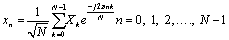(1)

where, xn is the n-th signal component in OFDM output symbol, Xk is the k-th data modulated symbol in OFDM frequency domain, and N is the number of subcarriers.

The PAPR (in dB) of the transmitted OFDM signal can be defined as :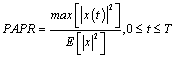(2)

Where E[·] is the expected value operator. The theoretical maximum of the PAPR for N number of subcarriers is as follows: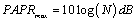(3)

PAPR is a random variable, because it is a function of the input data and the input data are random variable. Therefore PAPR can be calculated by using level crossing rate theorem that calculates the average number of times that the envelope of a signal crosses a given level. Knowing the amplitude distribution of the OFDM output signals, it is easy to compute the probability that the instantaneous amplitude will be above a given threshold and the same goes for power [12,13]. This is performed by calculating the Complementary cumulative distribution function (CCDF) for different PAPR. The CCDF of the original signal sequence’s PAPR above a threshold PAPR0 is written as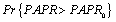. Thus for K statistical independent signal waveforms, CCDF can be rewritten as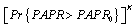, so that the probability of PAPR that exceeds the same threshold will drop to a small value. The probability of PAPR larger than a threshold z can be written as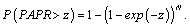Assume that M OFDM symbols carry the same information and that they are statistically independent of each other. In this case, the probability of PAPR greater than z is equals to the product of each independent candidate’s probability. This process can be written as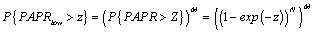(4)

Papr reduction by optimum-pts and SLM

Partial Transmit Sequence (PTS) algorithm was first proposed by Müller SH, Huber JB , which is a technique for improving the statistics of a multi-carrier signal. The basic idea of partial transmit sequences algorithm is to divide the original OFDM sequence intoQuadrature Phase Shift Keying several sub-sequences, and for each sub-sequence, multiplied by different weights until an optimum value is chosen [13-15]. Figure 1a is the block diagram of PTS algorithm. From the left side of diagram, we see that the data information in frequency domain X is separated into V non-overlapping sub-blocks and each sub-block vectors has the same size N .

Hence, we know that for every sub-block, it contains N/V nonzero elements and set the rest part to zero. Assume that these sub-blocks have the same size and no gap between each other, the sub-block vector is given by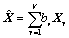(5)

where,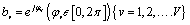is a weighting factor been used for phase rotation. The signal in time domain is obtained by applying IFFT operation on Xv, that is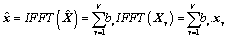(6)

Select one suitable factor combination b = [b1, b2,… bv,] which makes the result achieve optimum. The combination can be given by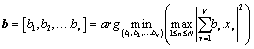(7)

where arg min (·) is the judgment condition that output the minimum value of function. In this way we can find the best b so as to optimize the PAPR performance. The additional cost we have to pay is the extra V-1 times IFFTs operation.

Figure 1b illustrates the basic structure of selected mapping method for suppressing the high PAPR. The key point of selected mapping method lies in how to generate multiple OFDM signals when the information is the same. First, defined different pseudo-random sequences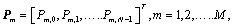where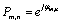and stands for the rotation factor. Pm,n is also known as the weighting factor. φm,n is uniformly distributed in [0 2π]. The N different subcarriers are modulated with these vectors respectively so as to generate candidate OFDM signals. This process can also be seen as performing dot product operation on a data block Xn with rotation factor Pm. In the reality, all the elements of phase sequence P1 are set to 1 so as to make this branch sequence the original signal. The symbols in branch m is expressed as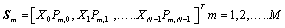(8)

and then transfer these M OFDM frames from frequency domain to time domain by performing IFFT calculation. The entire process is given by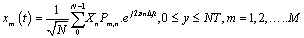(9)

Finally, the one which possess the smallest PAPR value is selected for transmission. Its mathematical expression is given as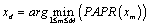(10)

where arg min(.) represent the argument of its value is minimized.

At the receiver, in order to correctly demodulate the received signal, it is necessary to know which sequence is linked to the smallest PAPR among M different candidates after performing the dot product. Hence, the receiver is required to learn information about selected phase vector sequence and ensure that the vector sequence is received correctly.

An intuitive approach is to select the whole sequence of branch number m as side information transmitted to the receiving end. However, in practice, the process does not necessarily require the delivery of the entire vector sequence. It can be realized by sending the route number of the vector sequence instead. This is only possible when the receiving end is able to restore the random phase sequence Pm by means of look-up (Table 1) or any other method.

Simulation Parameter Value
System Bandwidth 100MHz
Number of Sub-blocks used in PTS 4
Number of branches used in SLM 4
Number of sub-carriers 128
Guard Interval Ratio 1/4
Simulation Model Baseband
Modulation Scheme QPSK
Over-Sampling Factor 8
Size of Antenna Array 4 x 4
Channel type Rayleigh channel
Simulation Tool Matlab version 7.14.0.739 (R2012a)

Table 1: Complete system parameters used in the simulation.

SM-MIMO-OFDM system with optimum-pts as papr reduction technique

In Figure 2 shows the system level block diagram of SMMIMO- OFDM system using PTS as the PAPR reduction technique. It consists of three major sections; OFDM transmitter section, PTS section and Antenna section. Here on each branch of MIMO-OFDM the PTS block is inserted so that the data on each branch is further partitioned into four sub-blocks. After multiplication of data with sub-optimum weighting factor (bm) IFFT is applied to obtain OFDM symbols. Then the transmitted symbols have minimum PAPR. The peak power optimization block is responsible for finding the best weighting factor that produces smaller PAPR using iterative method.

SM-MIMO-OFDM system with SLM as papr reduction technique

The system level block diagram of SM-MIMO-OFDM system using SLM as PAPR reduction technique is shown in Figure 3. It consists of three major sections; OFDM transmitter section, SLM section and Antenna section. This system uses SLM at the transmitter side. On each branch of MIMO-OFDM system SLM is inserted so that the data block is further divided into 4 branches. After the multiplication of data on each branch by the rotation factor Pm, IFFT is applied to obtain OFDM symbol. Then the symbol having the minimum PAPR is selected and transmitted.

This SM-MIMO-OFDM system uses four transmit antennas (NT = 4) as given in Figure 3. At the input, binary data is first modulated using QPSK modulator, where the output of the modulator is of the form X , X ,…, X [N −1] ,and divided between four branches using De-multiplexer. On each branch the data is first converted from a serial stream into a set of N parallel symbols. This N parallel branch is further divided into four branches to implement SLM. Finally the best symbol having low PAPR is selected and it is parallel to serial converted before feeding to the antenna. In Figure 4 shows the system level block diagram of SM-MIMO-OFDM system receiver. The signals received from four different antennas are processed independently. After parallel to serial conversion data from all branches are multiplexed together. This multiplexed data is QPSK demodulated after Zero-Forcing equalization to recover the transmitted data.

Simulation and results

In Figure 5 shows the simulation result of using SLM and PTS method to an SM-MIMO-OFDM system, separately.

Figure 5: Comparison of PAPR reduction performances between PTS algorithm and SLM algorithm for SM-MIMO-OFDM system.

Based on the theory, we know that the IFFT calculation amount of these two methods is same when V = M, but for PTS method, it can provide more signal manifestations, thus, PTS method provide a superior performance on PAPR reduction. In fact, this deduction is confirmed by simulation result. From the Figure 5, we learned that with the same CCDF probability 1%, the PAPR value equals to 7 dB when Optimum PTS is employed, while the PAPR raise up to 8.2 dB when SLM is employed under the same circumstance.

The BER performance of the SM-MIMO-OFDM system with Optimum-PTS and SLM as PAPR reduction technique can be improved by increasing the value of Eb/N0. The observation shows that, the probability of bit error decreases with increase in the value of Eb/N0. The noise source considered here is only the AWGN noise. From Figure 6, it is seen that error probability of 10-6 is achieved at Eb/N0 of around 25.5 dB with Optimum PTS and for SLM the same BER can be achieved at 26.5 dB.

Figure 6: BER performance of SM-MIMO-OFDM system in Rayleigh channel with Optimum-PTS and SLM as PAPR reduction technique.

#### Conclusion

This paper focuses on the performance analysis of PAPR reduction in 4×4 Spatially Multiplexed MIMO-OFDM System using SLM and PTS Techniques. It shows that PAPR of high antenna configuration employing 4 antennas at the transmitter and receiver in MIMOOFDM system is better than the PAPR of an OFDM system. Further, Optimum-PTS technique for PAPR reduction is much better than SLM. By using Optimum-PTS technique the PAPR value is reduced by 3.9 dB and BER reaches to 10-6 at SNR 25.5 dB by using 6 redundant bits. While by using SLM technique the PAPR value is reduced by 2.2 dB and BER reaches to 10-6 at SNR 26.5 dB by using 2 redundant bits.

From the discussion above, we can say that Optimum-PTS algorithm is more suitable if system can tolerate more redundant information; otherwise, SLM algorithm is more acceptable when complexity becomes the first considering factor. In brief, compromise will be made for a reliable system.

#### Acknowledgement

I would like to thank Assistant Professor Brajesh Mishra for his kind support to complete this research.

#### References

Citation: Poudel B, Mishra B (2016) Performance Analysis of PAPR Reduction in 4×4 Spatially Multiplexed MIMO-OFDM System using SLM and Optimum-PTS Techniques. J Telecommun Syst Manage 5: 123. Doi: 10.4172/2167-0919.1000123

Copyright: ©2016 Poudel B, et al. This is an open-access article distributed under the terms of the Creative Commons Attribution License, which permits unrestricted use, distribution, and reproduction in any medium, provided the original author and source are credited.

Select your language of interest to view the total content in your interested language

Paris, France

London, UK

### 6th International Conference on Wireless,Telecommunication&IoT

Barcelona, Spain
##### Recommended Journals
Viewmore
###### Article Usage
• Total views: 9953
• [From(publication date): 3-2016 - Jan 23, 2020]
• Breakdown by view type
• HTML page views: 9801Can't read the image? click here to refresh